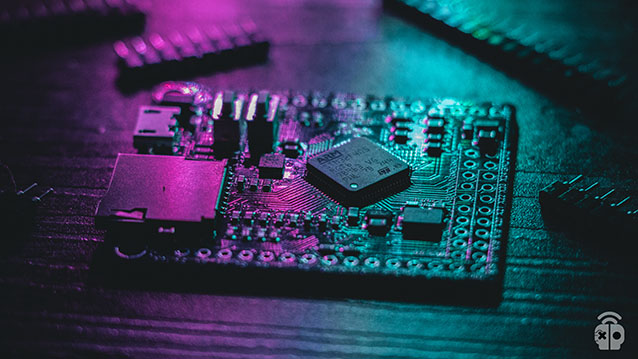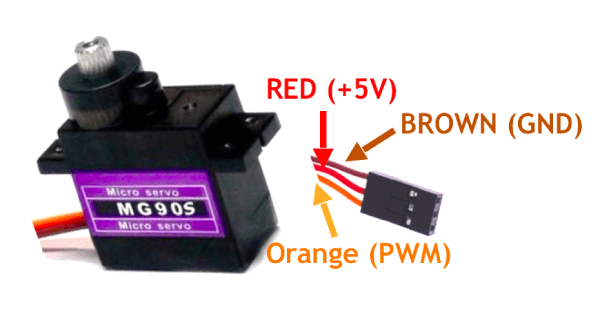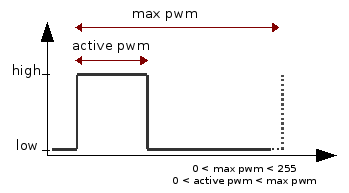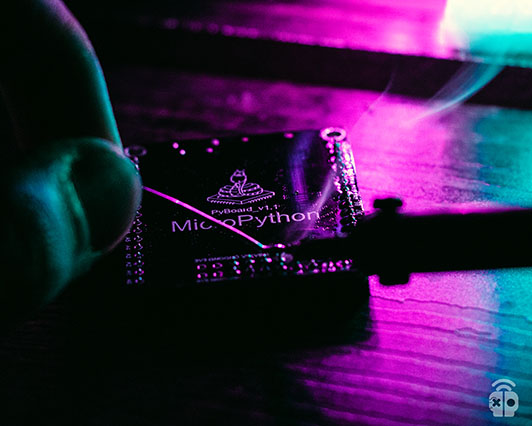-How to reach me CiferTech@gmail.comI’m currently working on IoT ProjectI’m currently learning Ai / MLAll my projects are available HERE!I regularly write ArticleAsk me about Nothing (Just kidding)Fun fact Earth is Not Flat# Control Servo SG90 With pyboard

## Category

In the previous tutorial, we covered the initial setup of pyboard and introduced some basic micro-python commands. In this tutorial, we are going to set up the SG90 servo motor. Micropython is one of the application programming languages for electronic hardware. On the other hand, ARM chips are completely suitable for this purpose and have a suitable operating speed. In addition to the economic price, they also have a higher productivity status.## Servo Motor

Standard three-wire servo motors “3wire hobby servo” have Ground, Vcc, and Signal bases, which can generally be controlled using PWM. In pyboard, for this purpose, we can use pins X1 to X4, which are actually our signal pins.## PWM concept in servo motor

Bandwidth modulation or PWM is a method to obtain a controllable and adjustable output voltage from a constant or variable input voltage. In other words, PWM is a term for a type of digital signal that is used in circuits for control. The microcontroller can produce two levels, HIGH and LOW, which are 5 and 0 volts, respectively, now if we need a voltage of 1.5 volts or 4 volts or any voltage between 0 and 5 volts, we use PWM.## Code

In this example, using a few lines of code, we can easily control the servo motor in question. Note that using Timer we can generate PWM and control the servo.

## MicroPython code analysis

Making servo objects; In the id section, the numbers 1 to 4 are placed, which specify the bases X1 to X4 of the pyboard board.

With this command, we specify the angle of the servo, which is specified by changing the angle, and also by equating time with the desired number, the amount of time it takes for the servo to reach the desired angle is specified. If time is not used, the servo will change to the set angle as soon as possible. It should be noted that the time is based on milliseconds.

In this command, the value of PWM pulse width is specified, which value will be placed instead of the value.

In the following command, the values can be changed as follows:

• pulse_min ==> Minimum allowed pulse width
• pulse_max ==> Maximum allowed pulse width
• pulse_centre ==>  Pulse width corresponding to zero position or servo origin
• pulse_angle_90 ==> Pulse width corresponding to an angle of 90 degrees
• pulse_speed_100 ==>pulse width corresponding to speed 100error: